Anzeige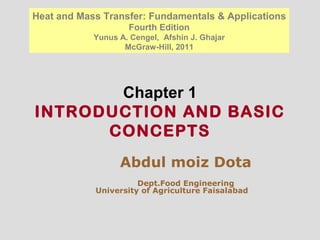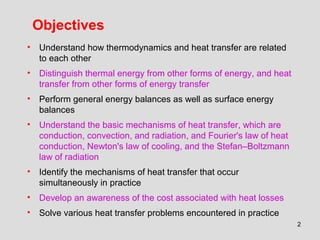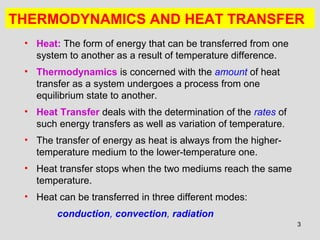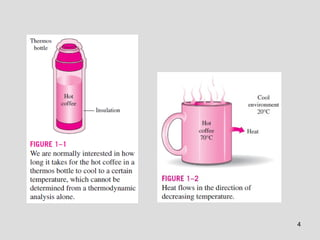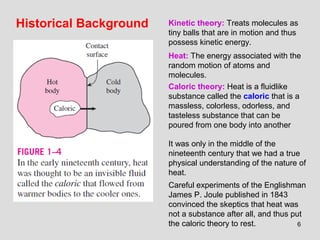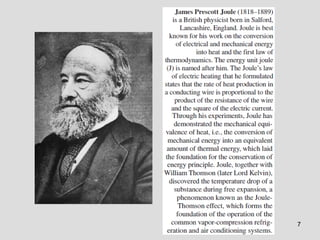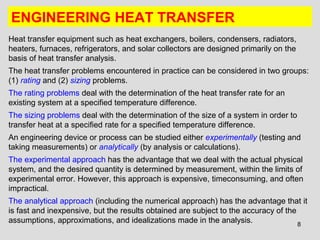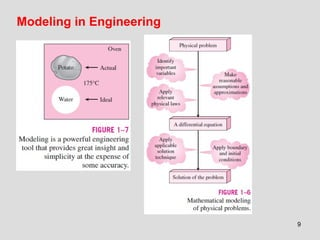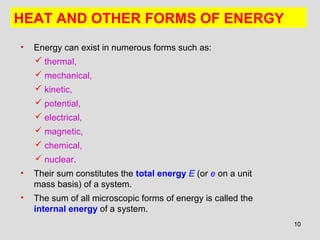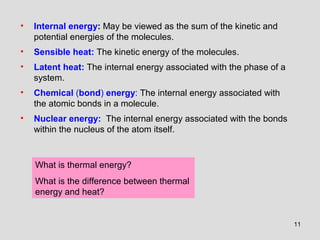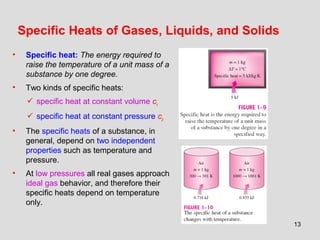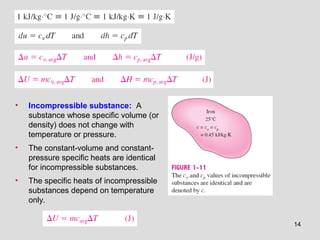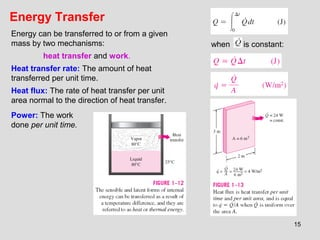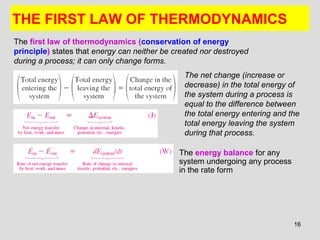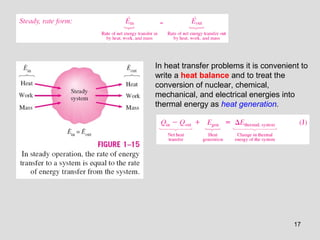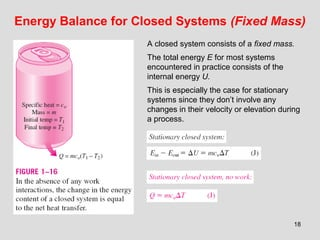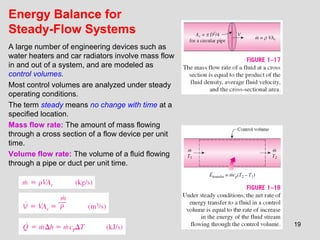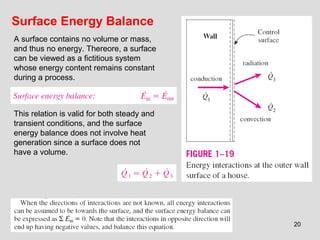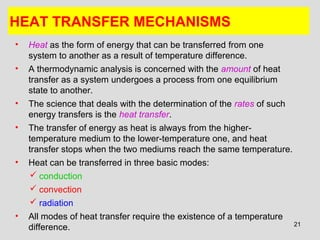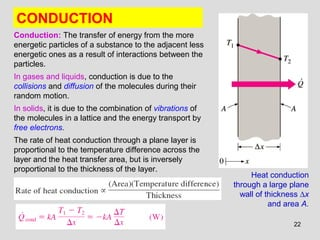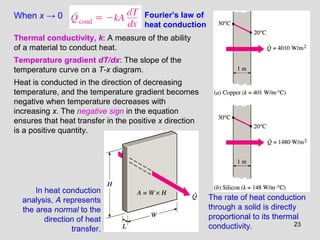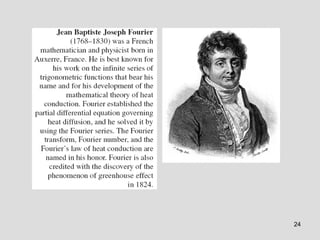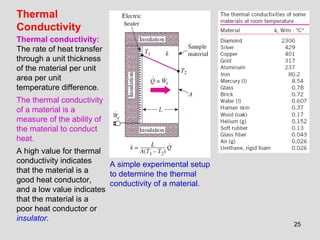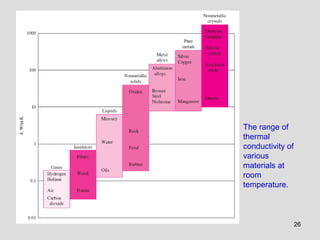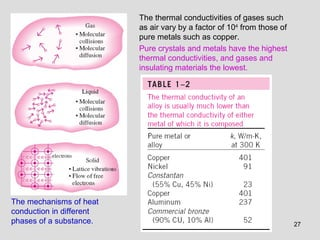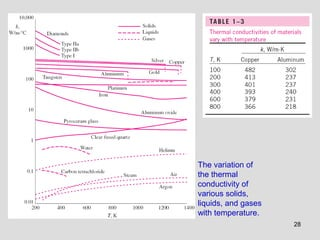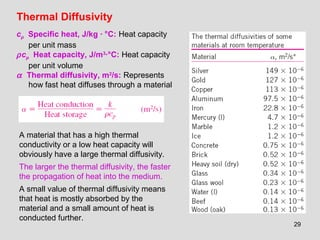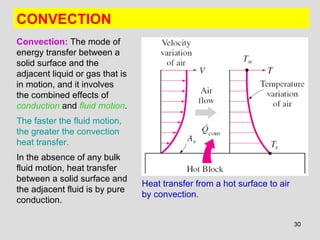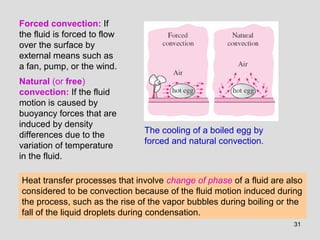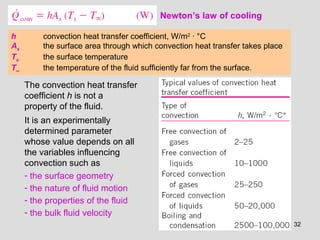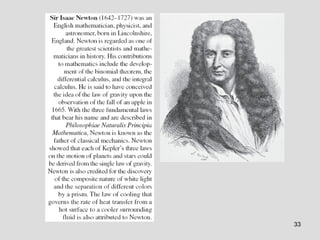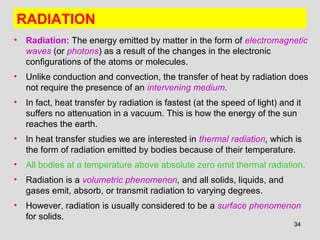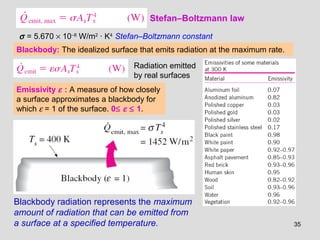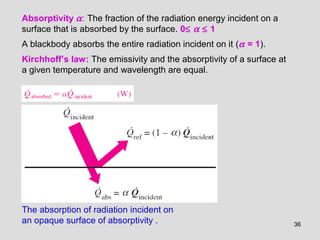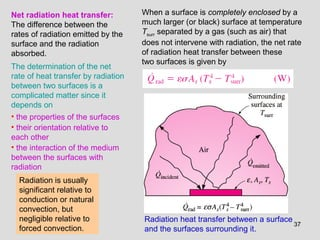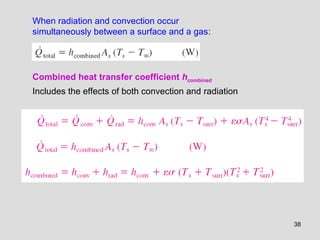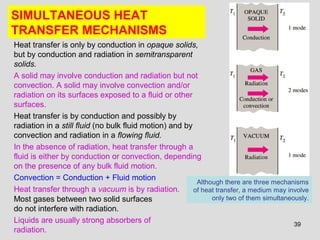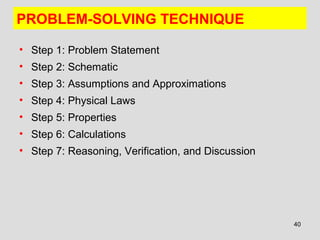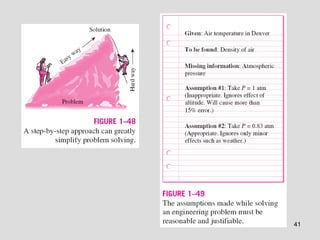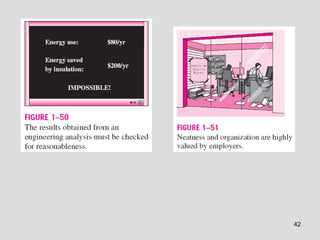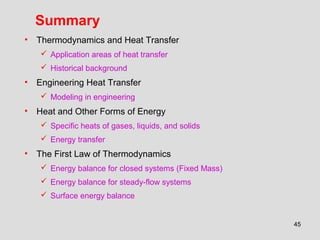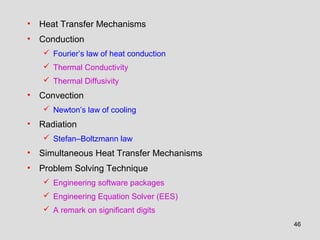1 von 46
Anzeige

### Chapter 1 INTRODUCTION AND BASIC CONCEPTS

1. Chapter 1 INTRODUCTION AND BASIC CONCEPTS Heat and Mass Transfer: Fundamentals & Applications Fourth Edition Yunus A. Cengel, Afshin J. Ghajar McGraw-Hill, 2011 Abdul moiz Dota Dept.Food Engineering University of Agriculture Faisalabad
2. 2 Objectives • Understand how thermodynamics and heat transfer are related to each other • Distinguish thermal energy from other forms of energy, and heat transfer from other forms of energy transfer • Perform general energy balances as well as surface energy balances • Understand the basic mechanisms of heat transfer, which are conduction, convection, and radiation, and Fourier's law of heat conduction, Newton's law of cooling, and the Stefan–Boltzmann law of radiation • Identify the mechanisms of heat transfer that occur simultaneously in practice • Develop an awareness of the cost associated with heat losses • Solve various heat transfer problems encountered in practice
3. 3 THERMODYNAMICS AND HEAT TRANSFER • Heat: The form of energy that can be transferred from one system to another as a result of temperature difference. • Thermodynamics is concerned with the amount of heat transfer as a system undergoes a process from one equilibrium state to another. • Heat Transfer deals with the determination of the rates of such energy transfers as well as variation of temperature. • The transfer of energy as heat is always from the higher- temperature medium to the lower-temperature one. • Heat transfer stops when the two mediums reach the same temperature. • Heat can be transferred in three different modes: conduction, convection, radiation
4. 4
5. 5 Application Areas of Heat Transfer 5
6. 6 Historical Background Kinetic theory: Treats molecules as tiny balls that are in motion and thus possess kinetic energy. Heat: The energy associated with the random motion of atoms and molecules. Caloric theory: Heat is a fluidlike substance called the caloric that is a massless, colorless, odorless, and tasteless substance that can be poured from one body into another It was only in the middle of the nineteenth century that we had a true physical understanding of the nature of heat. Careful experiments of the Englishman James P. Joule published in 1843 convinced the skeptics that heat was not a substance after all, and thus put the caloric theory to rest.
7. 7
8. 8 ENGINEERING HEAT TRANSFER Heat transfer equipment such as heat exchangers, boilers, condensers, radiators, heaters, furnaces, refrigerators, and solar collectors are designed primarily on the basis of heat transfer analysis. The heat transfer problems encountered in practice can be considered in two groups: (1) rating and (2) sizing problems. The rating problems deal with the determination of the heat transfer rate for an existing system at a specified temperature difference. The sizing problems deal with the determination of the size of a system in order to transfer heat at a specified rate for a specified temperature difference. An engineering device or process can be studied either experimentally (testing and taking measurements) or analytically (by analysis or calculations). The experimental approach has the advantage that we deal with the actual physical system, and the desired quantity is determined by measurement, within the limits of experimental error. However, this approach is expensive, timeconsuming, and often impractical. The analytical approach (including the numerical approach) has the advantage that it is fast and inexpensive, but the results obtained are subject to the accuracy of the assumptions, approximations, and idealizations made in the analysis.
9. 9 Modeling in Engineering
10. 10 • Energy can exist in numerous forms such as:  thermal,  mechanical,  kinetic,  potential,  electrical,  magnetic,  chemical,  nuclear. • Their sum constitutes the total energy E (or e on a unit mass basis) of a system. • The sum of all microscopic forms of energy is called the internal energy of a system. HEAT AND OTHER FORMS OF ENERGY
11. 11 • Internal energy: May be viewed as the sum of the kinetic and potential energies of the molecules. • Sensible heat: The kinetic energy of the molecules. • Latent heat: The internal energy associated with the phase of a system. • Chemical (bond) energy: The internal energy associated with the atomic bonds in a molecule. • Nuclear energy: The internal energy associated with the bonds within the nucleus of the atom itself. What is thermal energy? What is the difference between thermal energy and heat?
12. 12 Internal Energy and Enthalpy • In the analysis of systems that involve fluid flow, we frequently encounter the combination of properties u and Pv. • The combination is defined as enthalpy (h = u + Pv). • The term Pv represents the flow energy of the fluid (also called the flow work).
13. 13 Specific Heats of Gases, Liquids, and Solids • Specific heat: The energy required to raise the temperature of a unit mass of a substance by one degree. • Two kinds of specific heats:  specific heat at constant volume cv  specific heat at constant pressure cp • The specific heats of a substance, in general, depend on two independent properties such as temperature and pressure. • At low pressures all real gases approach ideal gas behavior, and therefore their specific heats depend on temperature only.
14. 14 • Incompressible substance: A substance whose specific volume (or density) does not change with temperature or pressure. • The constant-volume and constant- pressure specific heats are identical for incompressible substances. • The specific heats of incompressible substances depend on temperature only.
15. 15 Energy Transfer Energy can be transferred to or from a given mass by two mechanisms: heat transfer and work. Heat transfer rate: The amount of heat transferred per unit time. Heat flux: The rate of heat transfer per unit area normal to the direction of heat transfer. when is constant: Power: The work done per unit time.
16. 16 THE FIRST LAW OF THERMODYNAMICS The energy balance for any system undergoing any process in the rate form The first law of thermodynamics (conservation of energy principle) states that energy can neither be created nor destroyed during a process; it can only change forms. The net change (increase or decrease) in the total energy of the system during a process is equal to the difference between the total energy entering and the total energy leaving the system during that process.
17. 17 In heat transfer problems it is convenient to write a heat balance and to treat the conversion of nuclear, chemical, mechanical, and electrical energies into thermal energy as heat generation.
18. 18 Energy Balance for Closed Systems (Fixed Mass) A closed system consists of a fixed mass. The total energy E for most systems encountered in practice consists of the internal energy U. This is especially the case for stationary systems since they don’t involve any changes in their velocity or elevation during a process.
19. 19 Energy Balance for Steady-Flow Systems A large number of engineering devices such as water heaters and car radiators involve mass flow in and out of a system, and are modeled as control volumes. Most control volumes are analyzed under steady operating conditions. The term steady means no change with time at a specified location. Mass flow rate: The amount of mass flowing through a cross section of a flow device per unit time. Volume flow rate: The volume of a fluid flowing through a pipe or duct per unit time.
20. 20 Surface Energy Balance This relation is valid for both steady and transient conditions, and the surface energy balance does not involve heat generation since a surface does not have a volume. A surface contains no volume or mass, and thus no energy. Thereore, a surface can be viewed as a fictitious system whose energy content remains constant during a process.
21. 21 HEAT TRANSFER MECHANISMS • Heat as the form of energy that can be transferred from one system to another as a result of temperature difference. • A thermodynamic analysis is concerned with the amount of heat transfer as a system undergoes a process from one equilibrium state to another. • The science that deals with the determination of the rates of such energy transfers is the heat transfer. • The transfer of energy as heat is always from the higher- temperature medium to the lower-temperature one, and heat transfer stops when the two mediums reach the same temperature. • Heat can be transferred in three basic modes:  conduction  convection  radiation • All modes of heat transfer require the existence of a temperature difference.
22. 22 Heat conduction through a large plane wall of thickness ∆x and area A. CONDUCTION Conduction: The transfer of energy from the more energetic particles of a substance to the adjacent less energetic ones as a result of interactions between the particles. In gases and liquids, conduction is due to the collisions and diffusion of the molecules during their random motion. In solids, it is due to the combination of vibrations of the molecules in a lattice and the energy transport by free electrons. The rate of heat conduction through a plane layer is proportional to the temperature difference across the layer and the heat transfer area, but is inversely proportional to the thickness of the layer.
23. 23 When x → 0 Fourier’s law of heat conduction Thermal conductivity, k: A measure of the ability of a material to conduct heat. Temperature gradient dT/dx: The slope of the temperature curve on a T-x diagram. Heat is conducted in the direction of decreasing temperature, and the temperature gradient becomes negative when temperature decreases with increasing x. The negative sign in the equation ensures that heat transfer in the positive x direction is a positive quantity. The rate of heat conduction through a solid is directly proportional to its thermal conductivity. In heat conduction analysis, A represents the area normal to the direction of heat transfer.
24. 24
25. 25 Thermal Conductivity Thermal conductivity: The rate of heat transfer through a unit thickness of the material per unit area per unit temperature difference. The thermal conductivity of a material is a measure of the ability of the material to conduct heat. A high value for thermal conductivity indicates that the material is a good heat conductor, and a low value indicates that the material is a poor heat conductor or insulator. A simple experimental setup to determine the thermal conductivity of a material.
26. 26 The range of thermal conductivity of various materials at room temperature.
27. 27 The mechanisms of heat conduction in different phases of a substance. The thermal conductivities of gases such as air vary by a factor of 104 from those of pure metals such as copper. Pure crystals and metals have the highest thermal conductivities, and gases and insulating materials the lowest.
28. 28 The variation of the thermal conductivity of various solids, liquids, and gases with temperature.
29. 29 Thermal Diffusivity cp Specific heat, J/kg · °C: Heat capacity per unit mass ρcp Heat capacity, J/m3 ·°C: Heat capacity per unit volume α Thermal diffusivity, m2 /s: Represents how fast heat diffuses through a material A material that has a high thermal conductivity or a low heat capacity will obviously have a large thermal diffusivity. The larger the thermal diffusivity, the faster the propagation of heat into the medium. A small value of thermal diffusivity means that heat is mostly absorbed by the material and a small amount of heat is conducted further.
30. 30 CONVECTION Convection: The mode of energy transfer between a solid surface and the adjacent liquid or gas that is in motion, and it involves the combined effects of conduction and fluid motion. The faster the fluid motion, the greater the convection heat transfer. In the absence of any bulk fluid motion, heat transfer between a solid surface and the adjacent fluid is by pure conduction. Heat transfer from a hot surface to air by convection.
31. 31 Forced convection: If the fluid is forced to flow over the surface by external means such as a fan, pump, or the wind. Natural (or free) convection: If the fluid motion is caused by buoyancy forces that are induced by density differences due to the variation of temperature in the fluid. The cooling of a boiled egg by forced and natural convection. Heat transfer processes that involve change of phase of a fluid are also considered to be convection because of the fluid motion induced during the process, such as the rise of the vapor bubbles during boiling or the fall of the liquid droplets during condensation.
32. 32 Newton’s law of cooling h convection heat transfer coefficient, W/m2 · °C As the surface area through which convection heat transfer takes place Ts the surface temperature T∞ the temperature of the fluid sufficiently far from the surface. The convection heat transfer coefficient h is not a property of the fluid. It is an experimentally determined parameter whose value depends on all the variables influencing convection such as - the surface geometry - the nature of fluid motion - the properties of the fluid - the bulk fluid velocity
33. 33
34. 34 RADIATION • Radiation: The energy emitted by matter in the form of electromagnetic waves (or photons) as a result of the changes in the electronic configurations of the atoms or molecules. • Unlike conduction and convection, the transfer of heat by radiation does not require the presence of an intervening medium. • In fact, heat transfer by radiation is fastest (at the speed of light) and it suffers no attenuation in a vacuum. This is how the energy of the sun reaches the earth. • In heat transfer studies we are interested in thermal radiation, which is the form of radiation emitted by bodies because of their temperature. • All bodies at a temperature above absolute zero emit thermal radiation. • Radiation is a volumetric phenomenon, and all solids, liquids, and gases emit, absorb, or transmit radiation to varying degrees. • However, radiation is usually considered to be a surface phenomenon for solids.
35. 35 Stefan–Boltzmann law σ = 5.670 × 10−8 W/m2 · K4 Stefan–Boltzmann constant Blackbody: The idealized surface that emits radiation at the maximum rate. Blackbody radiation represents the maximum amount of radiation that can be emitted from a surface at a specified temperature. Emissivity ε : A measure of how closely a surface approximates a blackbody for which ε = 1 of the surface. 0≤ ε ≤ 1. Radiation emitted by real surfaces
36. 36 Absorptivity α: The fraction of the radiation energy incident on a surface that is absorbed by the surface. 0≤ α ≤ 1 A blackbody absorbs the entire radiation incident on it (α = 1). Kirchhoff’s law: The emissivity and the absorptivity of a surface at a given temperature and wavelength are equal. The absorption of radiation incident on an opaque surface of absorptivity .
37. 37 Radiation heat transfer between a surface and the surfaces surrounding it. Net radiation heat transfer: The difference between the rates of radiation emitted by the surface and the radiation absorbed. The determination of the net rate of heat transfer by radiation between two surfaces is a complicated matter since it depends on • the properties of the surfaces • their orientation relative to each other • the interaction of the medium between the surfaces with radiation Radiation is usually significant relative to conduction or natural convection, but negligible relative to forced convection. When a surface is completely enclosed by a much larger (or black) surface at temperature Tsurr separated by a gas (such as air) that does not intervene with radiation, the net rate of radiation heat transfer between these two surfaces is given by
38. 38 Combined heat transfer coefficient hcombined Includes the effects of both convection and radiation When radiation and convection occur simultaneously between a surface and a gas:
39. 39 SIMULTANEOUS HEAT TRANSFER MECHANISMS Although there are three mechanisms of heat transfer, a medium may involve only two of them simultaneously. Heat transfer is only by conduction in opaque solids, but by conduction and radiation in semitransparent solids. A solid may involve conduction and radiation but not convection. A solid may involve convection and/or radiation on its surfaces exposed to a fluid or other surfaces. Heat transfer is by conduction and possibly by radiation in a still fluid (no bulk fluid motion) and by convection and radiation in a flowing fluid. In the absence of radiation, heat transfer through a fluid is either by conduction or convection, depending on the presence of any bulk fluid motion. Convection = Conduction + Fluid motion Heat transfer through a vacuum is by radiation. Most gases between two solid surfaces do not interfere with radiation. Liquids are usually strong absorbers of radiation.
40. 40 PROBLEM-SOLVING TECHNIQUE • Step 1: Problem Statement • Step 2: Schematic • Step 3: Assumptions and Approximations • Step 4: Physical Laws • Step 5: Properties • Step 6: Calculations • Step 7: Reasoning, Verification, and Discussion
41. 41
42. 42
43. 43 EES (Engineering Equation Solver) (Pronounced as ease): EES is a program that solves systems of linear or nonlinear algebraic or differential equations numerically. It has a large library of built-in thermodynamic property functions as well as mathematical functions. Unlike some software packages, EES does not solve engineering problems; it only solves the equations supplied by the user. Engineering Software Packages Thinking that a person who can use the engineering software packages without proper training on fundamentals can practice engineering is like thinking that a person who can use a wrench can work as a car mechanic.
44. 44 A Remark on Significant Digits In engineering calculations, the information given is not known to more than a certain number of significant digits, usually three digits. Consequently, the results obtained cannot possibly be accurate to more significant digits. Reporting results in more significant digits implies greater accuracy than exists, and it should be avoided. A result with more significant digits than that of given data falsely implies more accuracy.
45. 45 Summary • Thermodynamics and Heat Transfer  Application areas of heat transfer  Historical background • Engineering Heat Transfer  Modeling in engineering • Heat and Other Forms of Energy  Specific heats of gases, liquids, and solids  Energy transfer • The First Law of Thermodynamics  Energy balance for closed systems (Fixed Mass)  Energy balance for steady-flow systems  Surface energy balance
46. 46 • Heat Transfer Mechanisms • Conduction  Fourier’s law of heat conduction  Thermal Conductivity  Thermal Diffusivity • Convection  Newton’s law of cooling • Radiation  Stefan–Boltzmann law • Simultaneous Heat Transfer Mechanisms • Problem Solving Technique  Engineering software packages  Engineering Equation Solver (EES)  A remark on significant digits
Anzeige